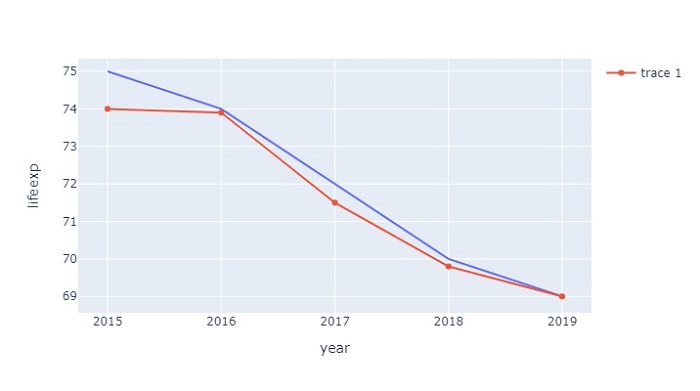# How to plot multiple lines on the same Y-axis in Python Plotly?

Plotly is an open-source plotting library in Python that can generate several different types interactive web-based visualizations that can be displayed in Jupyter notebooks, saved to standalone HTML files, or served as a part of web applications using Dash. Plotly can also be used in static document publishing and desktop editors such as PyCharm and Spyder.

In this tutorial, we will show how you can use Plotly to plot multiple lines on the same Y-axis on a chart. Here we will use plotly.express to generate figures. It contains a lot of methods to customize chart and render a chart into HTML format. In addition, we will use the Pandas module to generate the DataFrame.

Follow the steps given below to plot multiple lines on the same Y-axis.

Step 1

Import the plotly.express module and alias as px. Similarly, import the Pandas module and alias as pd.

import plotly.express as px
import pandas as pd


Step 2

Create a dataframe using the Pandas module.

data = {
'year':[2015,2016,2017,2018,2019],
'lifeexp':[75,74,72,70,69],
'state':['kerala','punjab','karnataka','andhra','odisha'],
'ratio':[74,73.9,71.5,69.8,69]
}
df = pd.DataFrame(data)


Step 3

Create a line plot using the px.line() method.

# Create line plot
fig = px.line(df, x=df['year'], y=df['lifeexp'])


Step 4

Use the add_scatter() method to create a scatter plot inside the figure.

fig.add_scatter(x=df['year'], y=df['ratio'])


## Example

The complete code to plot multiple line on same Y-axis follows,

import plotly.express as px
import pandas as pd

# Create dataset
data = {
'year':[2015,2016,2017,2018,2019],
'lifeexp':[75,74,72,70,69],
'state':['kerala','punjab','karnataka','andhra','odisha'],
'ratio':[74,73.9,71.5,69.8,69]
}

# Create dataframe
df = pd.DataFrame(data)

# Create Line plot
fig = px.line(df, x=df['year'], y=df['lifeexp'])